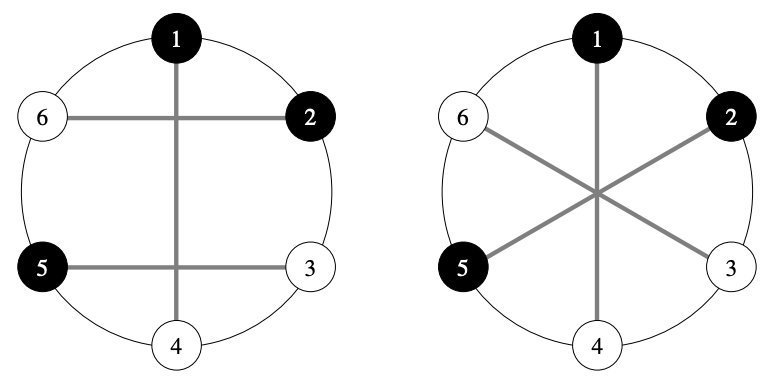시간 제한메모리 제한제출정답맞힌 사람정답 비율
1 초 512 MB219736.842%

## 문제

There are 2N points on a circle numbered from 1 through 2N, in clockwise order. Each point is either white or black. There are N white points and N black points.

We will draw N line segments connecting these points so that the following conditions are satisfied.

• Each point is an end point of exactly one line segment.
• Each line segment connects a white point and a black point.

Among the N line segments, the number of pairs of line segments intersecting each other is called the intersection number. Write a program which, given the information of the colors of the points, calculates the maximum of the intersection number when we draw N line segments.

## 입력

Read the following data from the standard input.

N S

Here S is a string of length 2N representing the colors of the points. Each character of S is either B or W, and the i-th character (1 ≤ i ≤ 2N) is the color of the i-th point. It is B if the point is black, and W if the point is white.

## 출력

Write one line to the standard output. The output should contain the maximum of the intersection number when we draw N line segments satisfying the conditions.

## 제한

• 1 ≤ N ≤ 200 000.
• S is a string of length 2N which consists of B and W. The character B appears N times in the string S, and the character W appears N times in the string S.

번호배점제한
14

N ≤ 8.

221

N ≤ 300.

310

N ≤ 2 000.

465

## 예제 입력 1

3
BBWWBW


## 예제 출력 1

2


If we draw line segments as in the figure on the left, then the intersection number is 2. On the other hand, if we draw line segments as in the figure on the right, then the intersection number is 3, but the conditions in the task statement are not satisfied.## 예제 입력 2

5
BWBWBBWBWW


## 예제 출력 2

8


## 예제 입력 3

10
WBBBWBBWWBWWBWWBWBWB


## 예제 출력 3

41


## 예제 입력 4

16
WWWBWBBBBWWBWWBWWBBWWBBBWBBBWWBW


## 예제 출력 4

105


## 채점 및 기타 정보

• 예제는 채점하지 않는다.